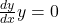Question

differentiate: y=152+26−37

in progress 0
2 months 2021-08-01T03:53:10+00:00 1 Answers 3 views 0General Formulas and Concepts:

Calculus

• The derivative of a constant is equal to 0

Step-by-step explanation:

Step 1: Define function

y = 152 + 26 – 37

Step 2: Simplify

Combine like terms

y = 141

Step 3: Find derivative

Derivative of any constant will be 0.